Roohnia, M., and Kohantorabi, M. (2015). "Dynamic methods to evaluate the shear modulus of wood," BioRes. 10(3), 4867-4876.

Abstract

There are different dynamic or static test methodologies for evaluating the shear and torsional moduli of wood. A preferred method is nondestructive, accurate, and standard. However, dynamic methods using conventional devices previously suggested for determining the orthotropic shear moduli of wood are neither accurate nor standard. Thus, a reliable method for evaluating the torsional modulus of wood through a torsional vibration test exists; however, it is not a standard test method at all. In this study, a standard methodology for refractory materials, ASTM C1548, was fitted to wood to meet the qualifications suggested in measuring the torsional vibration of an orthotropic material. A methodology based on Timoshenko’s advanced theory of free flexural vibration for orthotropic shear moduli was also defined to be compared to these reliable, standard procedures of torsional modulus evaluation. A promising conclusion was derived using improved computer-based instrumentation.

Dynamic Methods to Evaluate the Shear Modulus of Wood

Mehran Roohnia* and Mostafa Kohantorabi

There are different dynamic or static test methodologies for evaluating the shear and torsional moduli of wood. A preferred method is nondestructive, accurate, and standard. However, dynamic methods using conventional devices previously suggested for determining the orthotropic shear moduli of wood are neither accurate nor standard. Thus, a reliable method for evaluating the torsional modulus of wood through a torsional vibration test exists; however, it is not a standard test method at all. In this study, a standard methodology for refractory materials, ASTM C1548, was fitted to wood to meet the qualifications suggested in measuring the torsional vibration of an orthotropic material. A methodology based on Timoshenko’s advanced theory of free flexural vibration for orthotropic shear moduli was also defined to be compared to these reliable, standard procedures of torsional modulus evaluation. A promising conclusion was derived using improved computer-based instrumentation.

Keywords: Dynamic; Shear; Torsion; Vibration; Wood

Contact information: Department of Wood and Paper Science and Technology, College of Agriculture and Natural Resources, Karaj Branch, Islamic Azad University, Karaj, Iran;

*Corresponding author: mehran.roohnia@kiau.ac.ir

INTRODUCTION

The shear modulus is a mechanical property that indexes the ratio of stress to strain in shear deformation. For isotropic materials in which the direction of load to the material axis is neglected, the shear moduli oriented parallel to different coordinates are equal. In such a material, the shear modulus has been able to be substituted by the torsional modulus in many discussions.

For wood, an orthotropic material in which the relative conformity with an axis (i.e., longitudinal, radial, or tangential orientation) is of importance, the torsional modulus cannot easily be replaced by the shear modulus but appears as another material property related to a combination of orthotropic shear moduli. Based on theory, an effective shear modulus (comparable to the torsional modulus) may be calculated from both the orthotropic radial and tangential shear moduli (Bodig and Jayne 1989) (Eq. 1),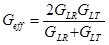(1)

where, Geff is the effective torsional modulus; GLR and GLT are two orthotropic radial and tangential shear moduli, respectively.

There are some static or dynamic well-known test methodologies for evaluating the shear moduli of wood. ASTM D198 (2003) is a standard methodology for evaluating the torsional modulus and the orthotropic shear moduli of wood in a static bending and torsion test method. The specimen is tested in one direction, and then must be bent in its second flexural direction to obtain the shear moduli in two orthotropic planes. Then, a torsion test is done on the same specimen to evaluate the torsional modulus. Reaching the proportional limit of deformation in this testing procedure can cause deficiencies in the specimen by which the second, third, or fourth cycle of tests could be affected. Harrison (2006) compared three testing methods for shear modulus assessment of machine stress-rated lumber according to ASTM standard D198 (2003). The average shear moduli results were significantly different. The shear moduli results determined from the two standard test methods (ASTM D198 three-point bending and torsion) and the five-point bending method, all of which were assumed to be equivalent, were significantly different. The five-point bending test had been proven effective in determining the shear properties of structural wood and wood-based composites (Janowiak et al. 2001).

Searching for methodologies causing fewer deficiencies in wood specimens through nondestructive procedures identified three other methodologies: Timoshenko’s advanced theorem of flexural vibration (for estimating the orthotropic shear moduli in a flexural vibration test) (Timoshenko 1954); ASTM standard C1548 (2012) (for evaluating the torsional modulus in a torsional vibration test; originally for refractory materials, but fitted to wood); and the formula provided by Divos et al. (1998) (for evaluating the torsional modulus in a torsional vibration test).

Divos (1998) compared the flexural vibration test (simultaneous evaluation of modulus of elasticity and shear moduli), static bending test (with variable span method), and torsional vibration test (Hearmon 1966; Perstorper 1994). They described the complexity of their comparison methodology concerning the shear stress distribution differences in bending and torsion. They highlighted that the surface of the specimen affects torsional evaluations while the core, around the neutral axis, could be of more importance in flexural static or dynamic evaluations. This difference was compensated for by using the clearest specimens to reach the highest possible level of soundness. They were also concerned with the relative error of determination. Thus, the instruments used at that time were not accurate enough to evaluate the modal frequency with a smaller error (0.5% relative error for frequency; compared to newer fast Fourier transform (FFT) analyzers, this is high). Divos et al. (1998) introduced torsional vibration as a reliable methodology for evaluating the torsional modulus in that paper but wished it were a standard test method. Nowadays, there are some standard test methods for materials other than wood that could be compared to Divos’ equation and fitted to wood. Divos also claimed that it was hard to distinguish the torsional mode in a mixture of flexural and torsional modes and that doing so requires experience. Industries (design codes), regardless to these difficulties, rely on an approximated shear modulus (G) value by dividing the modulus of elasticity (E) by 14 or 16. The E/G ratio is not always a constant value and varies from 8 to 24 (Divos et al. 1998; Kubojima et al.1997) or a bit bigger or smaller in some species. Kubojima and co-authors also reported the proper length/thickness ratios in terms of density, the E/G ratio (through the transcendental equation by Taylor’s series), and the mode number to minimize the error of the shear modulus determinations for some different species.

As mentioned by Harrison (2006), a comprehensive study of some of the tests used for shear moduli determination, including a quantitative and statistical comparison of the shear moduli values obtained by various different methods, would be beneficial to both the design community and the wood-based composites industry. Hence, this research aims to evaluate the shear moduli of solid wood specimens using reliable dynamic test methods. Three outstanding dynamic test methods for determining shear moduli values will be compared to understand whether proper equality exists.

By comparing the results, two questions will be answered. First, is the simultaneous evaluation of the shear moduli along the longitudinal modulus of elasticity in the free flexural vibration approach more accurate and reliable than what it was before, using older instrumentation? Is it comparable to that of Divos’ torsional modulus? Second, can the methodology described in ASTM standard C1548 (2012) be expanded to wood? Is it comparable to that of Divos’ torsional modulus?

EXPERIMENTAL

Materials

Clear specimens of Scots pine (Pinus sylvestris) were prepared in accordance with ISO standard 3129. The nominal dimensions were 40×16×400 mm (R×T×L) (wide rectangular specimens). Each specimen was further divided into two pieces 16×16×400 mm (R×T×L) in size (square section specimens). Twenty-five specimens were cut from large pine lumber, and the best 12 were selected. The selected specimens were clear and parallel grain. According to the ISO 3129 standard, the growth rings on the end surfaces of test pieces shall be parallel to one pair of opposite faces and perpendicular to the other pair while the adjacent faces are at right angles. So, the specimens from the older annual growth rings (i.e. the mature wood) were acceptable.

Methods

Flexural vibration test

Timoshenko (1954) improved upon the Euler-Bernoulli’s elementary flexural theory, taking into account shear deflection and rotary inertia. Bordonné (1989) introduced a solution to estimating the modulus of elasticity and the shear modulus simultaneously through a set of equations, fitting a linear trend to at least three points and referring to three consecutive initial modal frequencies of free flexural vibration of the both-ends-free beam. The slope is the ratio of E/G and the intercept refers to the specific modulus (Eq. 2),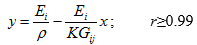(2)

where Ei is the modulus of elasticity parallel to the longitudinal axis of the beam, and Gij is the orthotropic shear modulus parallel to the neutral plane of flexural vibration.  is density, and K is a shape factor equal to 5/6 for a prismatic beam (Brancheriau and Bailleres 2002). The correlation coefficient (r) shows the coincidence of these at least three data points with the fitted linear trend that for an isotropic material the highest possible correlation coefficient is expected. Data points from modal frequencies have been introduced in previous literature (Bordonné 1989; Brancheriau and Bailleres 2002; Roohnia et al. 2010, 2011) as follows: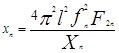(3)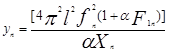(4)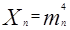(5)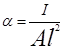(6)

In these equations, I is the moment of inertia, A is the cross sectional area, l is the length of the specimen, fn is the frequency of the nth mode of vibration, obtained from FFT spectrum, and mn is a constant due to the boundary condition of a beam and the mode number of the flexural vibration. In equations 3 and 4, F1n and F2n are calculated as follows: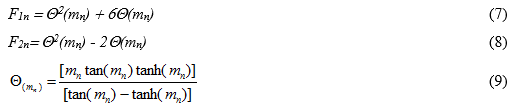An NDTlab® portable system (Roohnia 2007), registered in Iran, was used for FFT analysis to evaluate the dynamic properties (Fig. 1) from the modal frequencies. To simulate the free-free beam, the specimens were leaned on soft, thin pieces of rubber at their nodal points (0.224×length from each end). The specimen was excited at one end, and the vibration sound was recorded from the other end using a unidirectional microphone. The sampling rate of the recording software was 44100 Hz with a frequency resolution close to 3 Hz (the first frequency was close to 700 Hz). The relative repeatability error for frequency was measured to be less than 0.1% using 50 similar repetitions on the same specimen.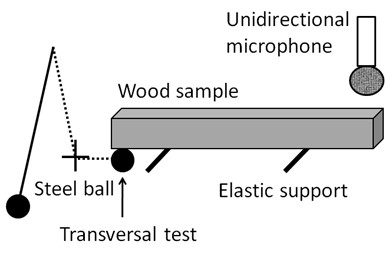Fig. 1. A both-ends-free beam under free flexural vibration

Torsional vibration test

VibraTor software, a new release of the NDTLAB® package (Roohnia 2007), was used to evaluate the torsional modes. The specimen leans on a cross-like, soft, thin piece of rubber (Fig. 2). A tap with a hammer excites the beam, causing torsional vibration. To recognize torsional modes from a mixture of torsional and flexural modes in the FFT spectrum, the tap and recording are done near the nodal points of the first mode of flexural vibration. The spectra in Fig. 3 show that this flexural mode is eliminated and the recognition of the torsional mode is slightly easier.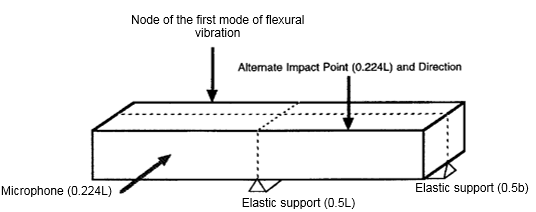Fig. 2. A free-free beam in free torsional vibration (ASTM C1548)

The sampling rate of the recording software was similar to that of the flexural vibration device. The torsional modulus can be evaluated using Divos’ suggested equation (Eq. 10) from the first mode of free torsional vibration,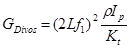(10)

where, f1 is the first torsional natural frequency; Ip is the polar moment of inertia (Ix + Iy). Kt is the result of c×a×b3 (a ≥ b); a and b are the cross-sectional dimensions; and c is a constant obtained from Table 1 (Divos et al. 1998).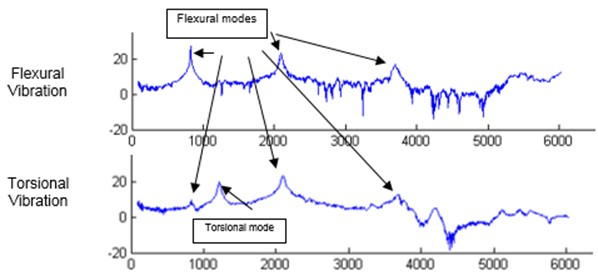Fig. 3. A comparison of the flexural and torsional FFT spectra of the same specimen (horizontal axis is frequency in Hz and vertical is amplitude in dB)

Table 1. The Constant c and the Aspect Ratio (a/b) (Divos et al. 1998)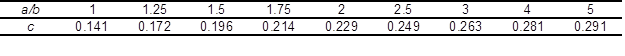There is also an ASTM standard methodology, albeit for refractory materials, that is hoped to be sufficiently comparable to wood testing methodologies. ASTM standard C1548 (2012) provides an equation for evaluating the torsional modulus from the first mode of free torsional vibration (Eq. 11).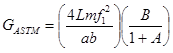(11)

where,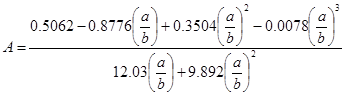(12)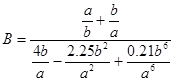(13)

Considering the above methods, the torsional moduli of the specimens were evaluated three times: in the flexural vibration by a combination of orthotropic shear moduli; using Divos’ suggested equation for free torsional vibration of a free-free beam; and using the ASTM standard C1548 (2012) methodology for the free torsional vibration of a free-free beam.

The results were compared and the effect of the testing method on the evaluated values was statistically studied.

RESULTS AND DISCUSSION

Before a statistical study of the effects of specimen widths and the testing method on the shear and torsional moduli is presented, the raw data as well as the scatter plots of the gathered data are shown in Table 2 and the following charts.

As Divos’ torsional modulus obtained from the torsional vibration is reported as reliable (Divos et al.1998), data analysis was done by comparing the test results for a wide range of specimens to those of obtained using Divos’s equation (Fig. 4). The equality of two methodologies for the torsional modulus (ASTM standard C1548 and Divos’ equations) is illustrated in Fig. 4a. The correlation coefficient of the relationship between ASTM standard C1548 and Divos’ method was very promising. Divos introduced his suggested torsional vibration as a sufficient methodology, but wished it were a certified, standard testing methodology. This equality, with the highest possible correlation coefficient, shows that the torsional vibration methodology described in ASTM standard C1548 for refractory materials could also be applicable to solid wood.

Table 2. The Raw Data of the Torsional Vibration of the Beams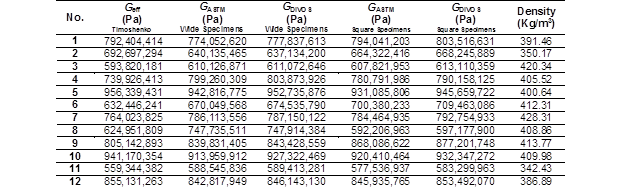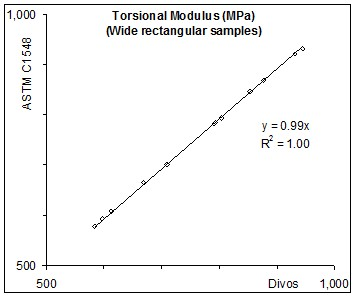a)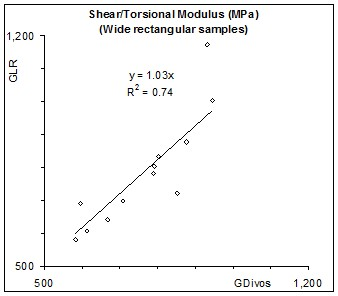b) Fig. 4. A comparison between different methods for evaluating the shear and torsional moduli in wide specimens

Figure 4b shows the radial shear modulus obtained using Timoshenko’s theory versus Divos’ torsional modulus. Greater values (by about 3%) for the radial shear modulus as compared to the total torsional modulus were expected; however, a better comparison could be obtained if a vertical direction of flexural vibration to determine the Timoshenko’s tangential shear modulus were possible in wide specimens.

To enable the specimens to be subjected to simultaneous determination of two orthotropic shear moduli, the specimens were divided into two similar square specimens for which the average of two values was taken into account for further analyses. Figure 5 shows these two orthotropic shear moduli. As expected, the tangential shear moduli were slightly smaller (by about 7%) than the radial ones. A combination of these moduli was calculated using Eq. 1 for the square specimens and was plotted against Divos’ torsional modulus of the wide specimens. A very small difference (of about 2%) was considered, but it is still too soon to comment on the significance of this difference. The specimen dimensions were altered (the width to the height ratio), which could have caused this difference.

The radial shear modulus was the only parameter obtained in both wide and square specimens. Using the same method of evaluation when the widths of the wide specimens were decreased to that of the heights (Fig. 7), the radial shear modulus was decreased to exactly the value seen in Fig. 6. This means that differences between the effective shear moduli and Divos’s torsional modulus could be ignored and, apart from the effects of specimen widths, equality between the methods was expected.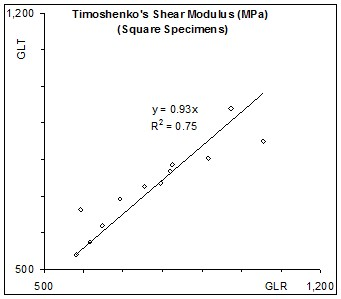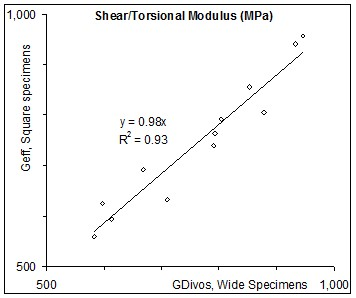Fig. 5. A comparison between two orthotropic shear moduli in square specimens Fig. 6. A comparison between effective shear modulus and Divos’s torsional modulus

The statistical analyses of variances to detect the effects of specimen width on the radial shear modulus evaluation showed that the 2% difference noted in Fig. 7 was not significant.

The effects of three different methodologies on the evaluated effective shear torsional moduli were also detected using variance analyses. The statistical test categorized all the results into a single, homogeneous subset. This means that, considering these particular test specimens, these three sets of values of effective shear and torsional moduli from Timoshenko’s advanced flexural theory, ASTM standard method C1548, and Divos’ torsional vibration test are statistically similar and reasonably substitutable in practice.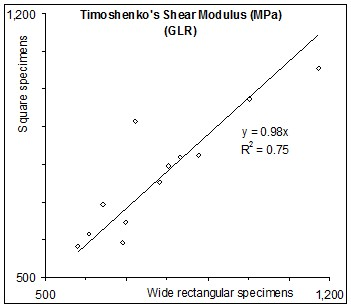Fig. 7. Effect of specimen width on the evaluated values of the radial shear moduli

The shear stress distribution differences in bending and torsion, considering the above obtained equality, promise a set of homogeneous specimens provided here in which the core and surfaces had equal qualities. For the inhomogeneous specimens in which the shell and core were different (e.g., case hardening defects in kiln drying), the equality of the flexural and torsional vibration tests, to obtain the shear and torsional moduli, were clearly expected to fade.

CONCLUSIONS

1. It was recognized that the torsional mode mixed with flexural modes of free vibration could be facilitated by omitting the flexural modes through appropriately placed tapping and recording on the nodes of the first flexural vibration mode.
2. ASTM standard C1548 (2012), originally for refractory materials, has similar capability to that of Divos’ methodology to reliably evaluate the torsional modulus of wood.
3. When a set of clear and homogeneous wood specimens was used, the effective shear modulus, a combination of two orthotropic radial and tangential shear moduli, obtained in the flexural vibration test was reasonably comparable with those determined by a torsional vibration test.
4. Considering the shear stress distribution differences in bending and torsion, the differences between the effective shear modulus in flexural vibration and the torsional modulus in torsional vibration is suggested to lead to a scenario to detect the inhomogeneity detection in core and shell of the lumber. Torsion is affected by the shell, while the bending horizontal shear is affected by the core of the flexural beam.

ACKNOWLEDGMENTS

The authors are grateful to the Islamic Azad University, Karaj Branch for supporting the current research project entitled “Nondestructive evaluation of shear moduli and strength of wood”.

REFERENCES CITED

ASTM C1548. (2012). “Standard test method for dynamic Young’s modulus, shear modulus and Poisson’s ratio of refractory materials by impulse excitation of vibration,” American Society of Testing and Materials, West Conshohocken, United states, 7pp.

ASTM D198. (2003). “Standard test methods of static tests of lumber in structural sizes,” West Conshohocken, United states, 21pp.

Bodig, J., and Jayne, B. A. (1989). Mechanics of Wood and Wood Composites (Persian Translation by G. Ebrahimi). Tehran University Press, Tehran.

Bordonné, P. A. (1989). “Module dynamique et frottement intérieur dans le bois: Mesures sur poutres flottantes en vibrations naturelles,” Ph.D Thesis, de l’INP de Lorraine soutenue à Nancy, France.

Brancheriau, L., and Baillères, H. (2002). “Natural vibration analysis of clear wooden beams: A theoretical review,” Wood Sci. Technol. 36(4), 347-365. DOI: 10.1007/s00226-002-0143-7

Divos, F., Tanaka, T., Nagao, H., and Kato, H. (1998). “Determination of shear modulus on construction size timber,” Wood Sci. Technol. 32(6), 393-402. DOI: 10.1007/bf00702796

Harrison, S. K. (2006). “Comparison of shear modulus test methods,” Master’s Thesis, Wood Science and Forest Products, Virginia Polytechnic and State University, Blacksburg, VA.

Hearmon, R. F. S. (1966). “Vibration testing of wood,” Forest Prod. J. 16(8), 29-39.

ISO (1975). “Wood- sampling methods and general requirements for physical and mechanical tests” – 1975-11-01- International Standard ISO 3129.

Janowiak, J. J., Hindman, D. P., Manbeck, H. B. (2001). “Orthotropic behavior of lumber composite materials,” Wood and Fiber Sci. 33(4), 580-594.

Kubojima, Y., Yhoshihara, H., Ohta, M., and Okano, T. (1997) “Accuracy of the shear modulus of wood obtained by Timoshenko’s theory of bending,” Mokuzai Gakkaishi 43(5) 439-443

Perstorper, M. (1994). “Quality of structural timber – End user requirements and performance control,” Ph.D. Thesis, Division of Steel and Timber Structures, Chalmers University of Technology, Göteborg, Sweden.

Roohnia, M. (2007). “NDT-LAB; System to evaluate the mechanical properties of wood,” Iranian Patent, IR-Patent No. 44032/22-08-1386.

Roohnia, M., Yavari A., and Tajdini, A. (2010). “Elastic parameters of poplar wood with end-cracks,” J. Ann. Forest Sci. 67(4), 409-409. DOI: 10.1051/forest/2009129

Roohnia, M., Tajdini, A., and Manouchehri, N. (2011). “Assessing wood in sounding boards considering the ratio of acoustical anisotropy,” NDT&E International 44(1), 13-20. DOI: 10.1016/j.ndteint.2010.09.001

Timoshenko, S. (1954). Vibration Problems in Engineering, Van Norstrand, New York.

Article submitted: February 27, 2015; Peer review completed: April 26, 2015; Revised version received: May 21, 2015; Accepted: June 14, 2015; Published: June 18, 2015.

DOI: 10.15376/biores.10.3.4867-4876